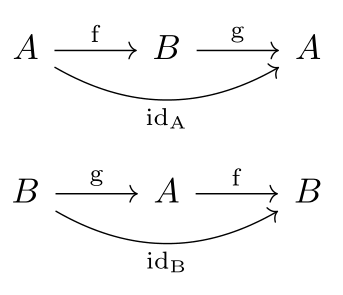Math and science::Algebra::Aluffi

# Injectivity and surjectivity

### Injective

A function $$f : A \to B$$ is injective iff

[$(\forall a' \in A)(\forall a'' \in A) \quad ?$]

Injections are often denoted like [$$A \quad ? \quad B$$].

### Surjective

A function $$f : A \to B$$ is surjective iff

[$(\forall b \in B) (\exists a \in A) \quad ?$]

Surjections are often denoted like [$$A \quad ? \quad B$$].

### Bijective

A function $$f$$ that is both injective and surjective is said to be bijective. A bijective function is called a bijection or a one-to-one correspondence or an isomorphism of sets; it is is often denoted like $$f : A \xrightarrow{\sim} B$$.

If there is a bijection between sets $$A$$ and $$B$$, then each element $$a$$ of $$A$$ can be matched with exactly one element $$b$$ of $$B$$. $$A$$ and $$B$$ are said to be [...]. The statement that $$A$$ and $$B$$ have such a relationship is often denoted as $$A \cong B$$.

### From the perspective of inverses

If $$f : A \to B$$ is a bijection, it can be 'flipped' to define a function $$g : B \to A$$. This is a flipping of $$\Gamma_f$$, the graph of $$f$$, along it's diagonal. $$f$$ being a bijection insures that such a flipping action produces a valid function. The function $$g$$ has two interesting properties:

[$g \circ f = \quad ?$]
[$f \circ g = \quad ?$]

These properties correspond to the statement that the following two diagrams compute:#### Bijections have inverses

The first identity above states that $$g$$ is a [...]-inverse of $$f$$. The second identity states that $$g$$ is a [...]-inverse of $$f$$. Combined we say that $$g$$ is the inverse of $$f$$, and denote it as [...]. Thus, bijections have inverses.

#### If a function has an inverse, is it a bijection?

This is [true or false?]. We can, in fact, break this statement into two pieces.

Let $$A$$ and $$B$$ be sets, with $$A \neq \emptyset$$, and let $$f : A \to B$$ be a function. Then the following bi-implications hold:

1. $$f$$ has a left-inverse if and only if it is [...].
2. $$f$$ has a right-inverse if and only if it is [...].

Can you think of the proof of these two bi-implications?

Futhermore, if $$f$$ has a left-inverse $$g_l$$ and right-inverse $$g_r$$, then these inverses must be the same function! This can be shown by considering:

[$g_l = \quad ? \quad = g_l (f g_r ) = (g_l f) g_r = \quad ? \quad = g_r$]